# 46+ Metric Conversion Chart Templates

A metric conversion chart is really useful when you cannot understand a particular measurement unit. For example, say a temperature is presented in Fahrenheit and you understand it in Celsius. In such situations, it’s the metric conversion chart for the temperature that will come in handy for you with its converted metric unit. This way, you have such charts for weight, capacity, length, distance and so on. These are useful for kids and hospitals to maintain such charts, especially height and weight conversion charts.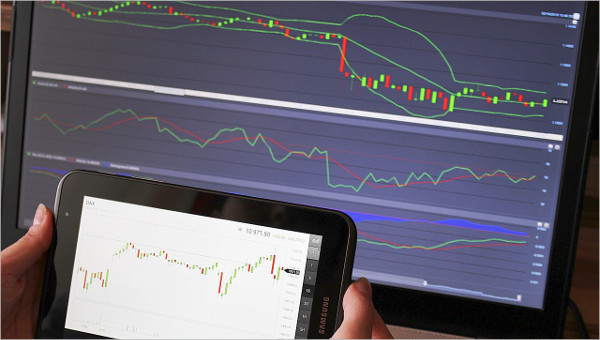## >Metric System Conversion Chart Templates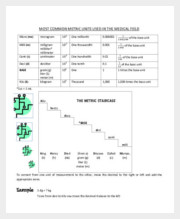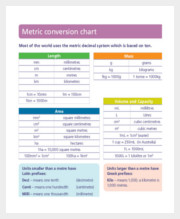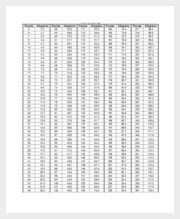A metric system conversion chart is one where you will have the converted metric of a particular measurement unit. It could be a conversion of kg to g or cm to inches and so on. The conversion charts are really useful when you cannot understand one particular metric unit and need to get another expression. [11+ Metric System Conversion Chart Templates]

## > Simple Metric Conversion Chart Templates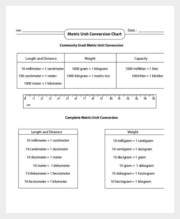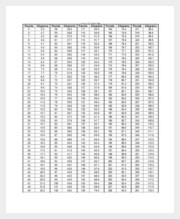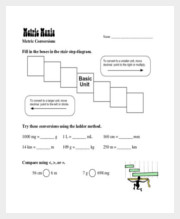A simple metric conversion chart is the one where you will usually have just one converted metric of the original metric- unlike the other elaborate charts where you will find several converted metrics of the original unit in question. These simple charts are good when you want to have a basic idea of a metric conversion chart. [7+ Simple Metric Conversion Chart Templates]

## > Basic Metric Conversion Chart Templates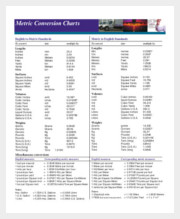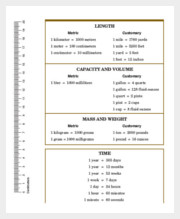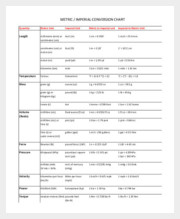If you are talking about basic metric conversion chart- well- mostly it’s the metric conversion chart for one definite attribute. The attribute could be distance or length or weight and so on. These basic charts are mostly for kids who need to have a preliminary understanding of the metric units and the converted measures. [8+ Basic Metric Conversion Chart Templates]

## > Metric Conversion Chart Templates For Kids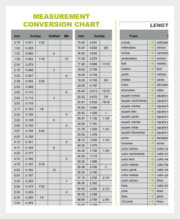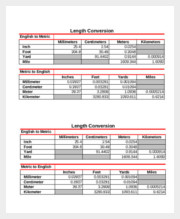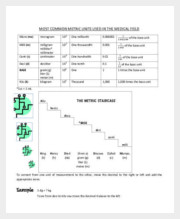No elementary math class is complete without metric conversion chart for kids. As soon as the little ones start to learn about the metric units, they must be taught about the other expressions of the same metric -so that they can have a comprehensive knowledge of measurements and their different expressions. [7+ Metric Conversion Chart Templates For Kids]

## > Metric Weight Conversion Chart Templates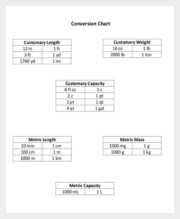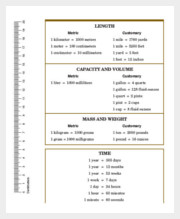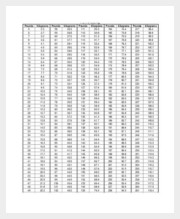When it comes to metric conversion charts, it’s the weight conversion charts that are most popular. In this case, you will have a typical metric unit of weight expressed in another variation or multiple variations. Thus, if the original metric unit was kg, the conversion chart might present it in pounds, stones and so on. [7+ Metric Weight Conversion Chart Templates]

## > Metric Unit Conversion Chart Templates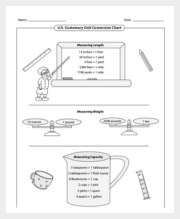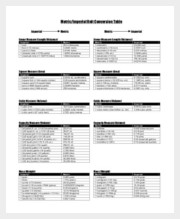Metric unit conversion charts can be many and varied. When it comes to measuring something and its expression in a certain way, it could be that some people are not really used to such expressions. Thus, there is the need of metric unit conversion charts which offer a certain measurement unit along with converted units – to ensure that the measurement is understood by all. [6+ Metric Unit Conversion Chart Templates]